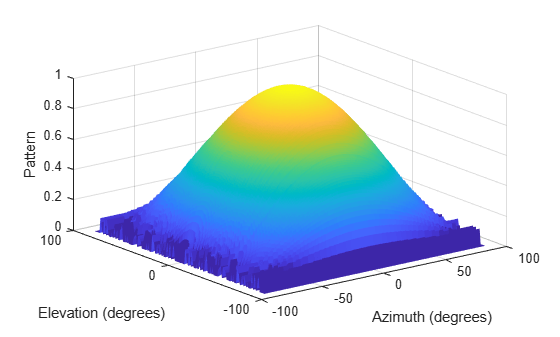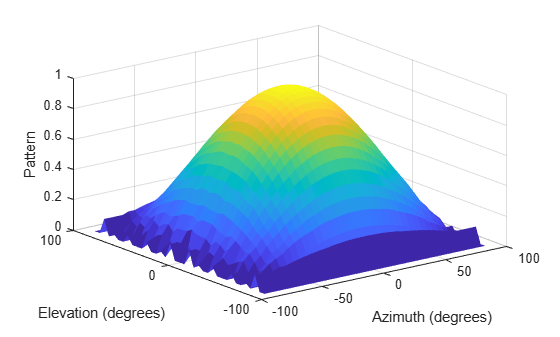# uv2azelpat

Convert radiation pattern from u/v form to azimuth/elevation form

## Syntax

``pat_azel = uv2azelpat(pat_uv,u,v)``
``pat_azel = uv2azelpat(pat_uv,u,v,az,el)``
``````[pat_azel,az_pat,el_pat] = uv2azelpat(___)``````

## Description

example

````pat_azel = uv2azelpat(pat_uv,u,v)` expresses the antenna radiation pattern `pat_azel` in azimuth/elevation angle coordinates instead of u/v space coordinates. `pat_uv` samples the pattern at u angles in `u` and v angles in `v`. The `pat_azel` matrix uses a default grid that covers azimuth values from –90 to 90 degrees and elevation values from –90 to 90 degrees. In this grid, `pat_azel` is uniformly sampled with a step size of 1 for azimuth and elevation. The function interpolates to estimate the response of the antenna at a given direction.```

example

````pat_azel = uv2azelpat(pat_uv,u,v,az,el)` uses vectors `az` and `el` to specify the grid at which to sample `pat_azel`. To avoid interpolation errors, `az` should cover the range [–90, 90] and `el` should cover the range [–90, 90].```

example

``````[pat_azel,az_pat,el_pat] = uv2azelpat(___)``` returns vectors containing the azimuth and elevation angles at which `pat_azel` samples the pattern, using any of the input arguments in the previous syntaxes.```

## Examples

collapse all

Convert a radiation pattern to azimuth/elevation form with the angles spaced 1° apart.

Define the pattern in terms of u and v. Because u and v values outside the unit circle are not physical, set the pattern values in this region to zero.

```u = -1:0.01:1; v = -1:0.01:1; [u_grid,v_grid] = meshgrid(u,v); pat_uv = sqrt(1 - u_grid.^2 - v_grid.^2); pat_uv(hypot(u_grid,v_grid) >= 1) = 0;```

Convert the pattern to azimuth/elevation space.

`pat_azel = uv2azelpat(pat_uv,u,v);`

Convert a radiation pattern to azimuth/elevation form with the angles spaced 1° apart.

Define the pattern in terms of u and v. Because u and v values outside the unit circle are not physical, set the pattern values in this region to zero.

```u = -1:0.01:1; v = -1:0.01:1; [u_grid,v_grid] = meshgrid(u,v); pat_uv = sqrt(1 - u_grid.^2 - v_grid.^2); pat_uv(hypot(u_grid,v_grid) >= 1) = 0;```

Convert the pattern to azimuth/elevation space. Store the azimuth and elevation angles for plotting.

`[pat_azel,az,el] = uv2azelpat(pat_uv,u,v);`

Plot the pattern.

```H = surf(az,el,pat_azel); H.LineStyle = 'none'; xlabel('Azimuth (degrees)') ylabel('Elevation (degrees)') zlabel('Pattern')```Convert a radiation pattern to azimuth/elevation form, with the angles spaced 5° apart.

Define the pattern in terms of u and v. Because u and v values outside the unit circle are not physical, set the pattern values in this region to zero.

```u = -1:0.01:1; v = -1:0.01:1; [u_grid,v_grid] = meshgrid(u,v); pat_uv = sqrt(1 - u_grid.^2 - v_grid.^2); pat_uv(hypot(u_grid,v_grid) >= 1) = 0;```

Define the set of azimuth and elevation angles at which to sample the pattern. Then convert the pattern.

```az = -90:5:90; el = -90:5:90; pat_azel = uv2azelpat(pat_uv,u,v,az,el);```

Plot the pattern.

```H = surf(az,el,pat_azel); H.LineStyle = 'none'; xlabel('Azimuth (degrees)') ylabel('Elevation (degrees)') zlabel('Pattern')```## Input Arguments

collapse all

Antenna radiation pattern in u/v form, specified as a Q-by-P matrix. `pat_uv` samples the 3-D magnitude pattern in decibels in terms of u and v coordinates. P is the length of the `u` vector and Q is the length of the `v` vector.

Data Types: `double`

u coordinates at which `pat_uv` samples the pattern, specified as a vector of length P. Each coordinate is between –1 and 1.

Data Types: `double`

v coordinates at which `pat_uv` samples the pattern, specified as a vector of length Q. Each coordinate is between –1 and 1.

Data Types: `double`

Azimuth angles at which `pat_azel` samples the pattern, specified as a vector of length L. Each azimuth angle is in degrees, between –90 and 90. Such azimuth angles are in the hemisphere for which u and v are defined.

Data Types: `double`

Elevation angles at which `pat_azel` samples the pattern, specified as a vector of length M. Each elevation angle is in degrees, between –90 and 90.

Data Types: `double`

## Output Arguments

collapse all

Antenna radiation pattern in azimuth-elevation coordinates, returned as a real-valued M-by-L matrix. `pat_azel` represents the magnitude pattern. L is the length of the `az_pat` vector, and M is the length of the `el_pat` vector. Units are in dB.

Azimuth angles at which the `pat_azel` output pattern is sampled, returned as a real-valued length-L vector. Units are in degrees.

Elevation angles at which the `pat_azel` output pattern is sampled, returned as a real-valued length-M vector. Units are in degrees.

collapse all

### U/V Space

The u and v coordinates are the direction cosines of a vector with respect to the y-axis and z-axis, respectively.

The u/v coordinates for the hemisphere x ≥ 0 are derived from the phi and theta angles, as follows:

`$\begin{array}{l}u=\mathrm{sin}\theta \mathrm{cos}\varphi \\ v=\mathrm{sin}\theta \mathrm{sin}\varphi \end{array}$`

In these expressions, φ and θ are the phi and theta angles, respectively.

To convert azimuth and elevation to u and v use the transformation

`$\begin{array}{l}u=\mathrm{cos}el\mathrm{sin}az\\ v=\mathrm{sin}el\end{array}$`

which is valid only in the range abs(az)≤=90.

The values of u and v satisfy the inequalities

`$\begin{array}{l}-1\le u\le 1\\ -1\le v\le 1\\ {u}^{2}+{v}^{2}\le 1\end{array}$`

Conversely, the phi and theta angles can be written in terms of u and v using

`$\begin{array}{l}\mathrm{tan}\varphi =v/u\\ \mathrm{sin}\theta =\sqrt{{u}^{2}+{v}^{2}}\end{array}$`

The azimuth and elevation angles can also be written in terms of u and v:

`$\begin{array}{l}\mathrm{sin}el=v\\ \mathrm{tan}az=\frac{u}{\sqrt{1-{u}^{2}-{v}^{2}}}\end{array}$`

### Phi Angle, Theta Angle

The phi angle (φ) is the angle from the positive y-axis to the vector’s orthogonal projection onto the yz plane. The angle is positive toward the positive z-axis. The phi angle is between 0 and 360 degrees. The theta angle (θ) is the angle from the x-axis to the vector itself. The angle is positive toward the yz plane. The theta angle is between 0 and 180 degrees.

The figure illustrates phi and theta for a vector that appears as a green solid line.The coordinate transformations between φ/θ and az/el are described by the following equations

`$\begin{array}{l}\mathrm{sin}el=\mathrm{sin}\varphi \mathrm{sin}\theta \\ \mathrm{tan}az=\mathrm{cos}\varphi \mathrm{tan}\theta \\ \mathrm{cos}\theta =\mathrm{cos}el\mathrm{cos}az\\ \mathrm{tan}\varphi =\mathrm{tan}el/\mathrm{sin}az\end{array}$`

### Azimuth Angle, Elevation Angle

The azimuth angle of a vector is the angle between the x-axis and the orthogonal projection of the vector onto the xy plane. The angle is positive in going from the x axis toward the y axis. Azimuth angles lie between –180 and 180 degrees. The elevation angle is the angle between the vector and its orthogonal projection onto the xy-plane. The angle is positive when going toward the positive z-axis from the xy plane. By default, the boresight direction of an element or array is aligned with the positive x-axis. The boresight direction is the direction of the main lobe of an element or array.

Note

The elevation angle is sometimes defined in the literature as the angle a vector makes with the positive z-axis. The MATLAB® and Phased Array System Toolbox™ products do not use this definition.

This figure illustrates the azimuth angle and elevation angle for a vector shown as a green solid line.